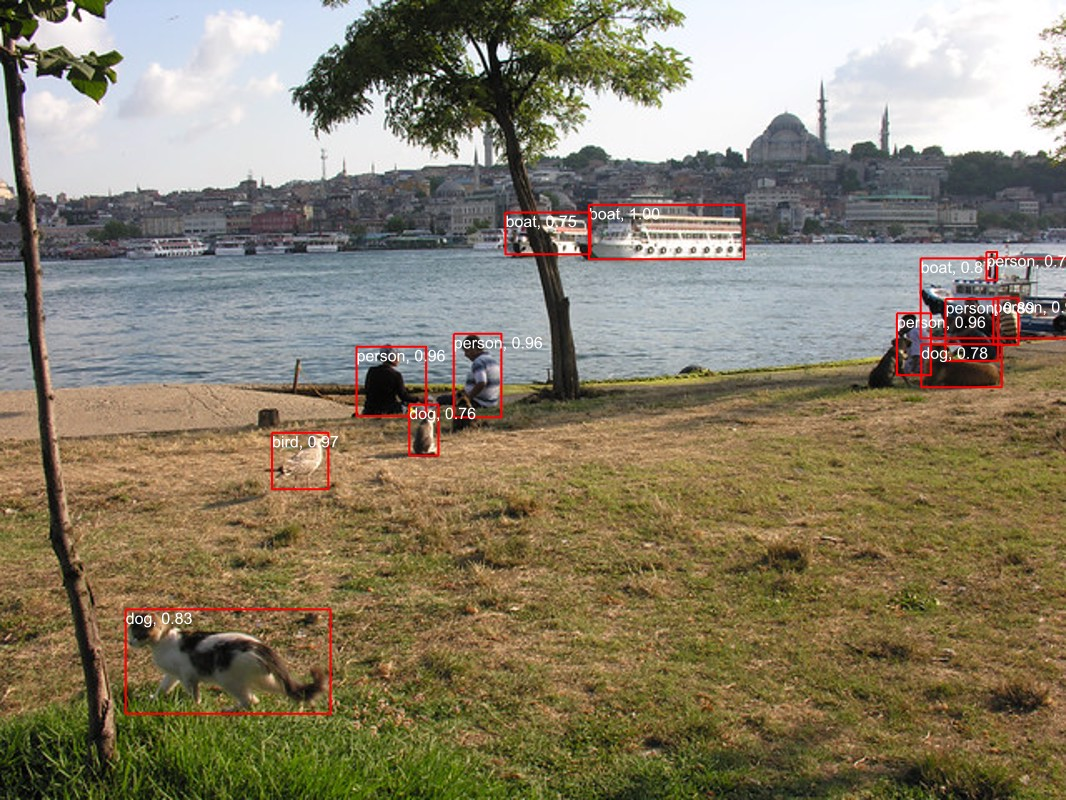# Object detection with Faster RCNN in C#

The sample walks through how to run a pretrained Faster R-CNN object detection ONNX model using the ONNX Runtime C# API.

The source code for this sample is available here.

## Prerequisites

To run this sample, you’ll need the following things:

1. Install .NET Core 3.1 or higher for you OS (Mac, Windows or Linux).
3. Download this demo image to test the model. You can also use any image you like.

## Get started

Now we have everything set up, we can start adding code to run the model on the image. We’ll do this in the main method of the program for simplicity.

Firstly, let’s read the path to the model, path to the image we want to test, and path to the output image:

``````string modelFilePath = args;
string imageFilePath = args;
string outImageFilePath = args;
``````

Next, we will read the image in using the cross-platform image library ImageSharp:

``````using Image<Rgb24> image = Image.Load<Rgb24>(imageFilePath, out IImageFormat format);
``````

Note, we’re specifically reading the `Rgb24` type so we can efficiently preprocess the image in a later step.

### Resize image

Next, we will resize the image to the appropriate size that the model is expecting; it is recommended to resize the image such that both height and width are within the range of [800, 1333].

``````float ratio = 800f / Math.Min(image.Width, image.Height);
using Stream imageStream = new MemoryStream();
image.Mutate(x => x.Resize((int)(ratio * image.Width), (int)(ratio * image.Height)));
image.Save(imageStream, format);
``````

### Preprocess image

Next, we will preprocess the image according to the requirements of the model:

``````var paddedHeight = (int)(Math.Ceiling(image.Height / 32f) * 32f);
var paddedWidth = (int)(Math.Ceiling(image.Width / 32f) * 32f);
var mean = new[] { 102.9801f, 115.9465f, 122.7717f };
for (int y = paddedHeight - image.Height; y < image.Height; y++)
{
Span<Rgb24> pixelSpan = image.GetPixelRowSpan(y);
for (int x = paddedWidth - image.Width; x < image.Width; x++)
{
input[0, y, x] = pixelSpan[x].B - mean;
input[1, y, x] = pixelSpan[x].G - mean;
input[2, y, x] = pixelSpan[x].R - mean;
}
}
``````

Here, we’re creating a Tensor of the required size `(channels, paddedHeight, paddedWidth)`, accessing the pixel values, preprocessing them and finally assigning them to the tensor at the appropriate indicies.

### Setup inputs

Next, we will create the inputs to the model:

``````var inputs = new List<NamedOnnxValue>
{
NamedOnnxValue.CreateFromTensor("image", input)
};
``````

To check the input node names for an ONNX model, you can use Netron to visualise the model and see input/output names. In this case, this model has `image` as the input node name.

### Run inference

Next, we will create an inference session and run the input through it:

``````using var session = new InferenceSession(modelFilePath);
``````

### Postprocess output

Next, we will need to postprocess the output to get boxes and associated label and confidence scores for each box:

``````var resultsArray = results.ToArray();
float[] boxes = resultsArray.AsEnumerable<float>().ToArray();
long[] labels = resultsArray.AsEnumerable<long>().ToArray();
float[] confidences = resultsArray.AsEnumerable<float>().ToArray();
var predictions = new List<Prediction>();
var minConfidence = 0.7f;
for (int i = 0; i < boxes.Length - 4; i += 4)
{
var index = i / 4;
if (confidences[index] >= minConfidence)
{
{
Box = new Box(boxes[i], boxes[i + 1], boxes[i + 2], boxes[i + 3]),
Label = LabelMap.Labels[labels[index]],
Confidence = confidences[index]
});
}
}
``````

Note, we’re only taking boxes that have a confidence above 0.7 to remove false positives.

### View prediction

Next, we’ll draw the boxes and associated labels and confidence scores on the image to see how the model went:

``````using var outputImage = File.OpenWrite(outImageFilePath);
Font font = SystemFonts.CreateFont("Arial", 16);
foreach (var p in predictions)
{
image.Mutate(x =>
{
x.DrawLines(Color.Red, 2f, new PointF[] {

new PointF(p.Box.Xmin, p.Box.Ymin),
new PointF(p.Box.Xmax, p.Box.Ymin),

new PointF(p.Box.Xmax, p.Box.Ymin),
new PointF(p.Box.Xmax, p.Box.Ymax),

new PointF(p.Box.Xmax, p.Box.Ymax),
new PointF(p.Box.Xmin, p.Box.Ymax),

new PointF(p.Box.Xmin, p.Box.Ymax),
new PointF(p.Box.Xmin, p.Box.Ymin)
});
x.DrawText(\$"{p.Label}, {p.Confidence:0.00}", font, Color.White, new PointF(p.Box.Xmin, p.Box.Ymin));
});
}
image.Save(outputImage, format);
``````

For each box prediction, we’re using ImageSharp to draw red lines to create the boxes, and drawing the label and confidence text.

## Running the program

Now the program is created, we can run it will the following command:

``````dotnet run [path-to-model] [path-to-image] [path-to-output-image]
``````

e.g. running:

``````dotnet run ~/Downloads/FasterRCNN-10.onnx ~/Downloads/demo.jpg ~/Downloads/out.jpg
``````

detects the following objects in the image: